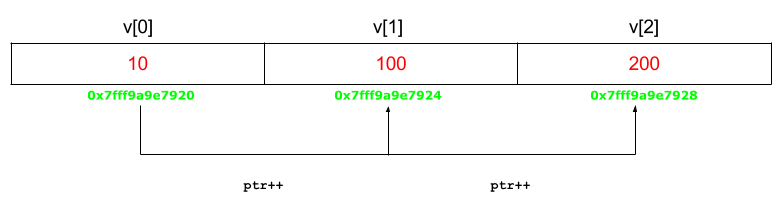# C program to sort an array using pointers

Given an array of size n, the task is to sort this array using pointers in C.

Examples:

```Input: n = 5, arr[] = {0, 23, 14, 12, 9}
Output: {0, 9, 12, 14, 23}

Input: n = 3, arr[] = {7, 0, 2}
Output: {0, 2, 7}
```

## Recommended: Please try your approach on {IDE} first, before moving on to the solution.

Approach: The array can be fetched with the help of pointers with the pointer variable pointing to the base address of the array. Hence in order to sort the array using pointers, we need to access the elements of the array using (pointer + index) format.Below is the implementation of the above approach:

Program:

 `#include ` ` `  `// Function to sort the numbers using pointers ` `void` `sort(``int` `n, ``int``* ptr) ` `{ ` `    ``int` `i, j, t; ` ` `  `    ``// Sort the numbers using pointers ` `    ``for` `(i = 0; i < n; i++) { ` ` `  `        ``for` `(j = i + 1; j < n; j++) { ` ` `  `            ``if` `(*(ptr + j) < *(ptr + i)) { ` ` `  `                ``t = *(ptr + i); ` `                ``*(ptr + i) = *(ptr + j); ` `                ``*(ptr + j) = t; ` `            ``} ` `        ``} ` `    ``} ` ` `  `    ``// print the numbers ` `    ``for` `(i = 0; i < n; i++) ` `        ``printf``(``"%d "``, *(ptr + i)); ` `} ` ` `  `// Driver code ` `int` `main() ` `{ ` `    ``int` `n = 5; ` `    ``int` `arr[] = { 0, 23, 14, 12, 9 }; ` ` `  `    ``sort(n, arr); ` ` `  `    ``return` `0; ` `} `

Output:

```0 9 12 14 23
```

My Personal Notes arrow_drop_upCheck out this Author's contributed articles.

If you like GeeksforGeeks and would like to contribute, you can also write an article using contribute.geeksforgeeks.org or mail your article to contribute@geeksforgeeks.org. See your article appearing on the GeeksforGeeks main page and help other Geeks.

Please Improve this article if you find anything incorrect by clicking on the "Improve Article" button below.

Article Tags :
Practice Tags :

2

Please write to us at contribute@geeksforgeeks.org to report any issue with the above content.